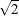# The NPAR1WAY Procedure

#### Multiple Comparisons Based on Pairwise Rankings

If you specify the DSCF option, PROC NPAR1WAY computes the Dwass, Steel, Critchlow-Fligner (DSCF) multiple comparison analysis, which is based on pairwise two-sample Wilcoxon comparisons (Dwass, 1960; Steel, 1960; Critchlow and Fligner, 1991). The DSCF analysis is available when the number of CLASS variable levels (samples) is greater than 2. There are r(r–1)/2 pairs of samples, where r is the total number of samples.

For each pair of samples, PROC NPAR1WAY ranks the observations and computes the standardized Wilcoxon test statistic, which is described in the sections Simple Linear Rank Tests for Two-Sample Data and Wilcoxon Scores. The procedure does not include a continuity correction in the Wilcoxon test statistics that it computes for the DSCF analysis. The DSCF statistic for a pair of samples is computed asz, where z is the two-sample standardized Wilcoxon statistic. Under the null hypothesis of no location differences among the r samples, the distribution of the DSCF statistics can be approximated by the studentized range distribution for r independent standard normal variables. The p-value for a two-sample DSCF comparison is the percentile of the studentized range distribution that corresponds to the value of the DSCF statistic. See Hollander and Wolfe (1999) and Juneau (2007, 2004) for more information.

When you specify the DSCF option, PROC NPAR1WAY displays the Pairwise Two-Sided Multiple Comparison Analysis table, which contains the following information for each two-sample comparison: comparison identification (two class variable levels), two-sample Wilcoxon test statistic, DSCF statistic, and two-sided DSCF p-value. You can store these statistics in a SAS data set by using the Output Delivery System (ODS). The ODS name for this table is DSCF. For more information, see the section ODS Table Names and Chapter 20: Using the Output Delivery System.